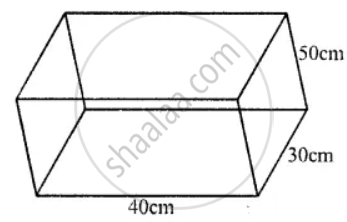# A Closed Box is Cuboid in Shape with Length = 40 Cm, Breadth = 30 Cm and Height = 50 Cm. It is Made of a Thin Metal Sheet. Find the Cost of Metal Sheet Required to Make 20 Such Boxes - Mathematics

Sum

A closed box is cuboid in shape with length = 40 cm, breadth = 30 cm and height = 50 cm. It is made of a thin metal sheet. Find the cost of metal sheet required to make 20 such boxes, if 1 m2 of metal sheet costs Rs. 45.

#### SolutionLength of closed box (l) = 40 cm

and height (h) = 50 cm

Total surface area = 2 (lb + bh + hl)

= 2 (40 × 30 + 30 × 50 + 50 × 40) cm2

= 2 (1200 + 1500 + 2000) cm2

= 2 × 4700

= 9400 cm2

Surface area of sheet used for 20 such boxes = 9400 × 20 = 188000 cm2

∴ 188000 cm2 = (188000)/(100 xx 100) = 18.8 " m"^2

Cost of 1 m2 sheet = Rs. 45

Total cost = ₹ 18.8 × 45 = ₹ 846

Is there an error in this question or solution?
Chapter 21: Surface Area, Volume and Capacity - Exercise 21 (A) [Page 238]

#### APPEARS IN

Selina Concise Mathematics Class 8 ICSE
Chapter 21 Surface Area, Volume and Capacity
Exercise 21 (A) | Q 11 | Page 238
Share